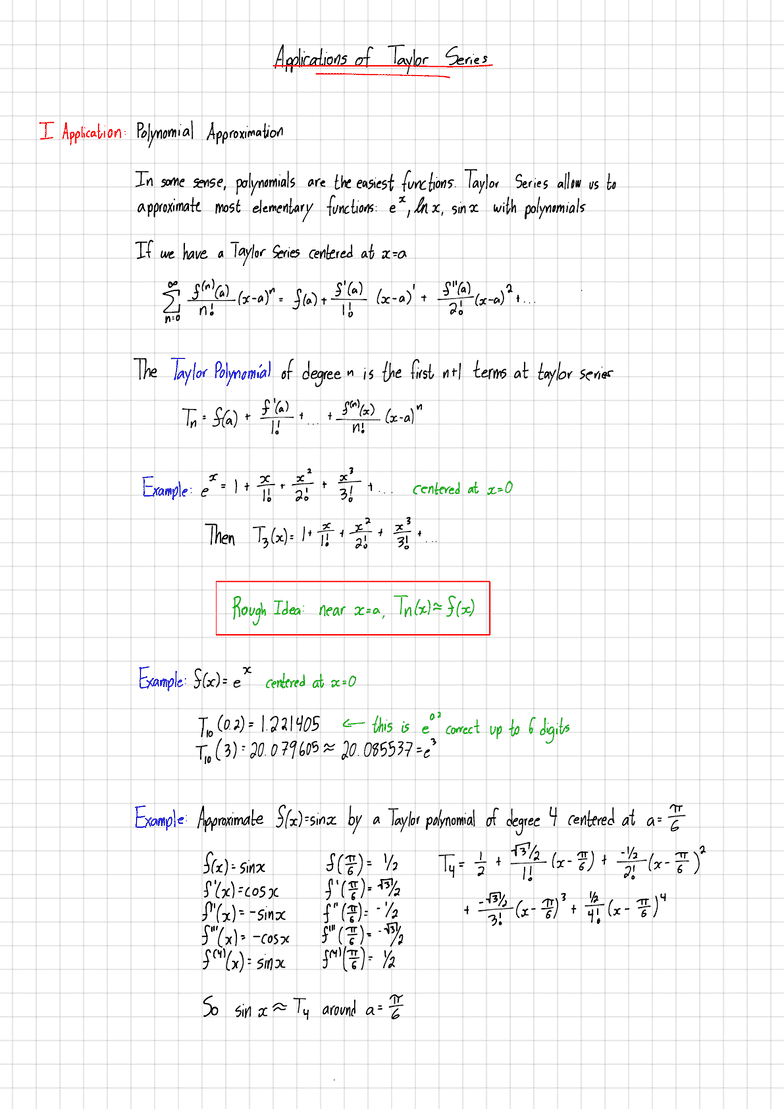Class Notes (1,100,000)
CA (620,000)
McMaster (50,000)
MATH (3,000)
Lecture 25

# MATH 1XX3 Lecture Notes - Lecture 25: Taylor Series

Department
Mathematics
Course Code
MATH 1XX3
Professor
Lecture
25

This preview shows half of the first page. to view the full 3 pages of the document.Applicationsoftaylorse
IApplication :Polynomial Approximation
In some sense ,polynomials are the easiest
functions .Taylor Series allow us to
approximate most elementary functions :ex ,lnx .sinx with polynomials
If we have aTaylor Series centered at x. -a
Fy ftp.dlx.at .fiat +5¥ lx .as
'
+fshIc*aPt ...
.
The Taylor Polynomial of degree in is the first NH terms at taylor series
Tn =Sta)+ftp.T +
...t.HN#Cx.al "
Example :et =I+TT '+¥t... centered at x=O
then T3H= HE +II +¥
,+
.. .
Rough Idea :near x=a .Tnlxkflx )
Example :Stock e
"
centered at x=O
I,o( 0.21=1.221405 this is e
"
correct up to 6digits
to (3) :20.
07.9605=20.085537 =e3
Example :Approximate flxtsinx by aTaylor polynomial of degree 4centered at a=ft
fact '
-sinx feat 42 Ty ='s 't T¥lx .F) +¥1 x-EP
ftxlicosx f'(Tt )=Abn
ft'bd= -sinx f"ftp.t -
'k+M¥4 -top the (x.It )"
f'''(x1= -cos ,c f'''(E) = 'MY,
FHYX):sinx fat #.Yz
So sin xat around a=E
.
###### You're Reading a Preview

Unlock to view full version